# CSCE 580 Artificial Intelligence Ch 3 Uninformed Blind

• Slides: 76
Download presentation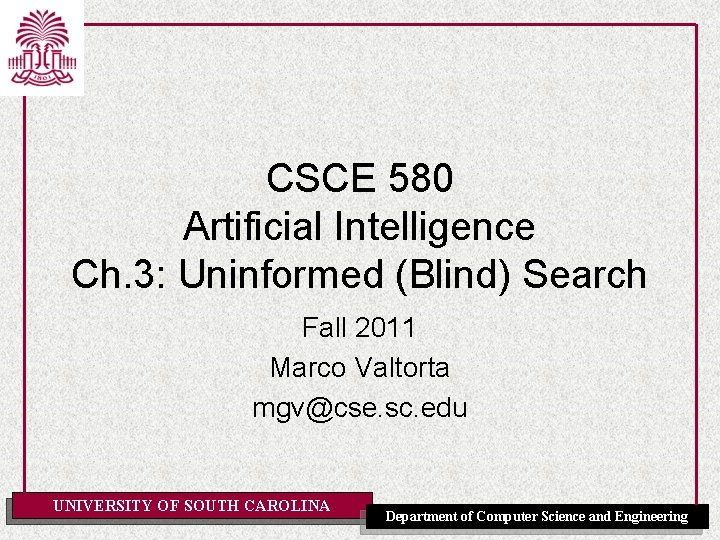CSCE 580 Artificial Intelligence Ch. 3: Uninformed (Blind) Search Fall 2011 Marco Valtorta [email protected] sc. edu UNIVERSITY OF SOUTH CAROLINA Department of Computer Science and Engineering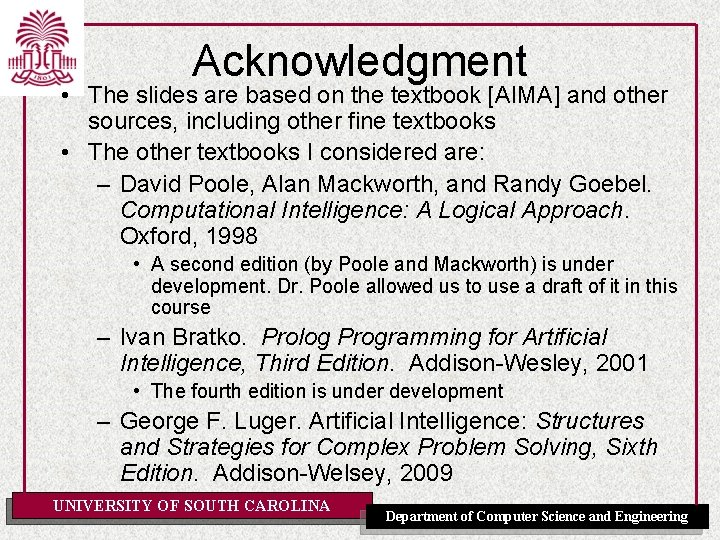Acknowledgment • The slides are based on the textbook [AIMA] and other sources, including other fine textbooks • The other textbooks I considered are: – David Poole, Alan Mackworth, and Randy Goebel. Computational Intelligence: A Logical Approach. Oxford, 1998 • A second edition (by Poole and Mackworth) is under development. Dr. Poole allowed us to use a draft of it in this course – Ivan Bratko. Prolog Programming for Artificial Intelligence, Third Edition. Addison-Wesley, 2001 • The fourth edition is under development – George F. Luger. Artificial Intelligence: Structures and Strategies for Complex Problem Solving, Sixth Edition. Addison-Welsey, 2009 UNIVERSITY OF SOUTH CAROLINA Department of Computer Science and EngineeringOutline • • • Problem-solving agents Problem types Problem formulation Example problems Basic search algorithms UNIVERSITY OF SOUTH CAROLINA Department of Computer Science and Engineering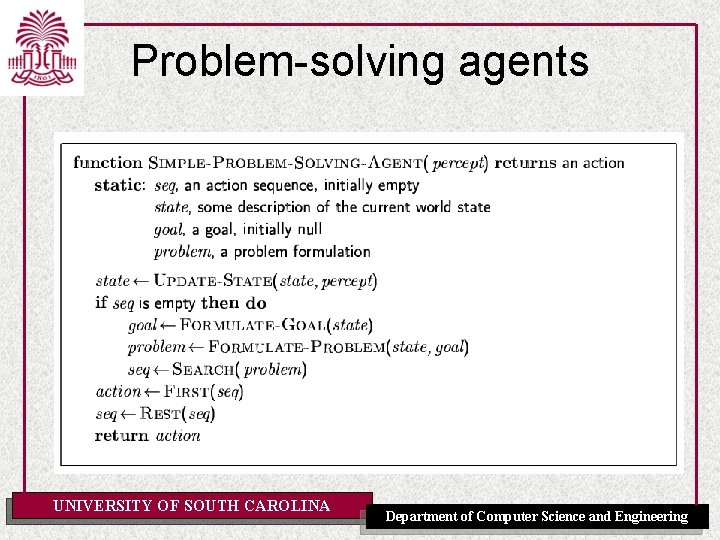Problem-solving agents UNIVERSITY OF SOUTH CAROLINA Department of Computer Science and EngineeringExample: Romania • On holiday in Romania; currently in Arad. • Flight leaves tomorrow from Bucharest • Formulate goal: – be in Bucharest • Formulate problem: – states: various cities – actions: drive between cities • Find solution: – sequence of cities, e. g. , Arad, Sibiu, Fagaras, Bucharest UNIVERSITY OF SOUTH CAROLINA Department of Computer Science and Engineering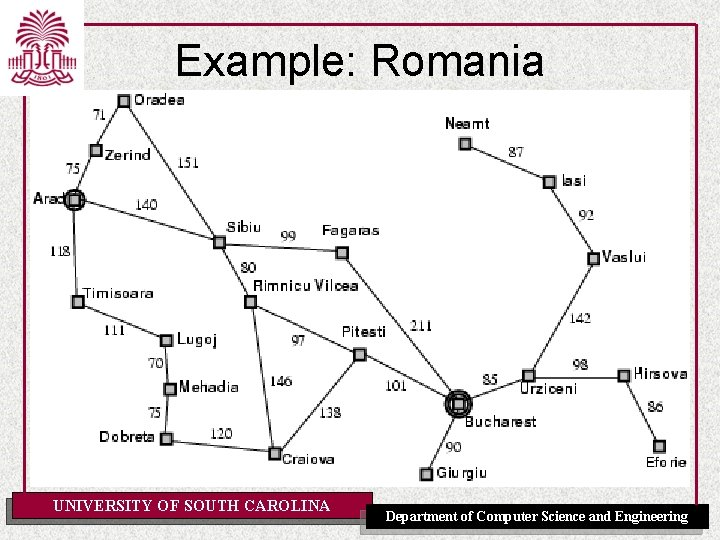Example: Romania UNIVERSITY OF SOUTH CAROLINA Department of Computer Science and Engineering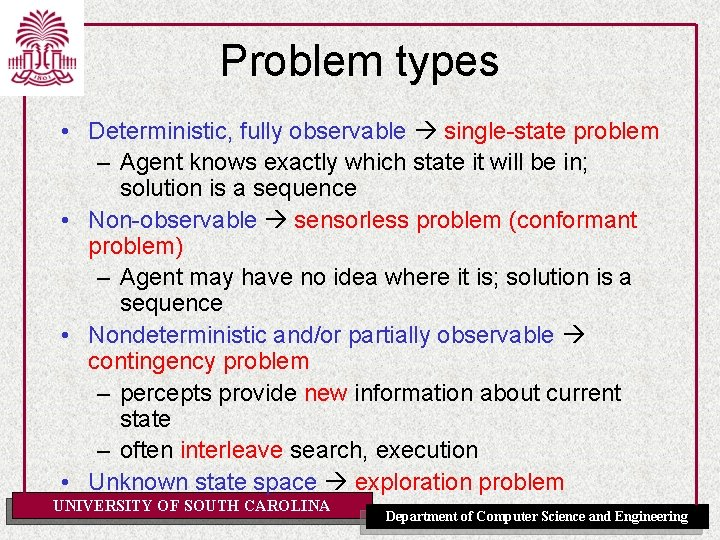Problem types • Deterministic, fully observable single-state problem – Agent knows exactly which state it will be in; solution is a sequence • Non-observable sensorless problem (conformant problem) – Agent may have no idea where it is; solution is a sequence • Nondeterministic and/or partially observable contingency problem – percepts provide new information about current state – often interleave search, execution • Unknown state space exploration problem UNIVERSITY OF SOUTH CAROLINA Department of Computer Science and Engineering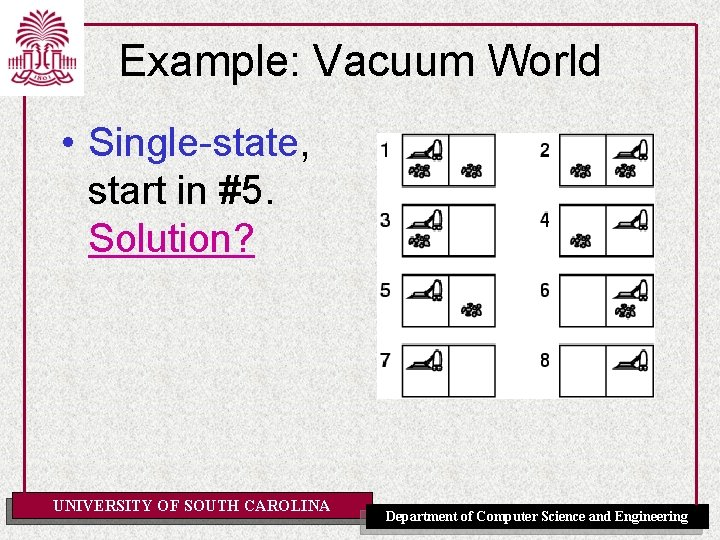Example: Vacuum World • Single-state, start in #5. Solution? UNIVERSITY OF SOUTH CAROLINA Department of Computer Science and Engineering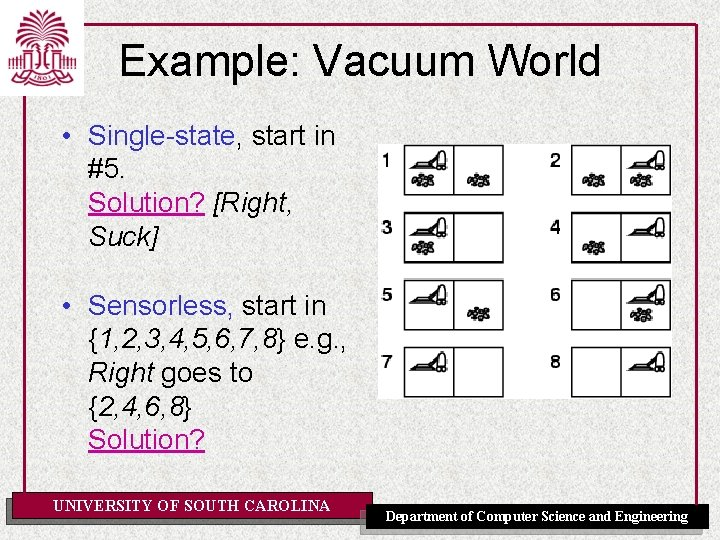Example: Vacuum World • Single-state, start in #5. Solution? [Right, Suck] • Sensorless, start in {1, 2, 3, 4, 5, 6, 7, 8} e. g. , Right goes to {2, 4, 6, 8} Solution? UNIVERSITY OF SOUTH CAROLINA Department of Computer Science and EngineeringExample: Vacuum World • Sensorless, start in {1, 2, 3, 4, 5, 6, 7, 8} e. g. , Right goes to {2, 4, 6, 8} Solution? [Right, Suck, Left, Suck] • Contingency – Nondeterministic: Suck may dirty a clean carpet – Partially observable: [location, dirt] at current location are the only percepts – Percept: [L, Clean], i. e. , start in #5 or #7 Solution? UNIVERSITY OF SOUTH CAROLINA Department of Computer Science and Engineering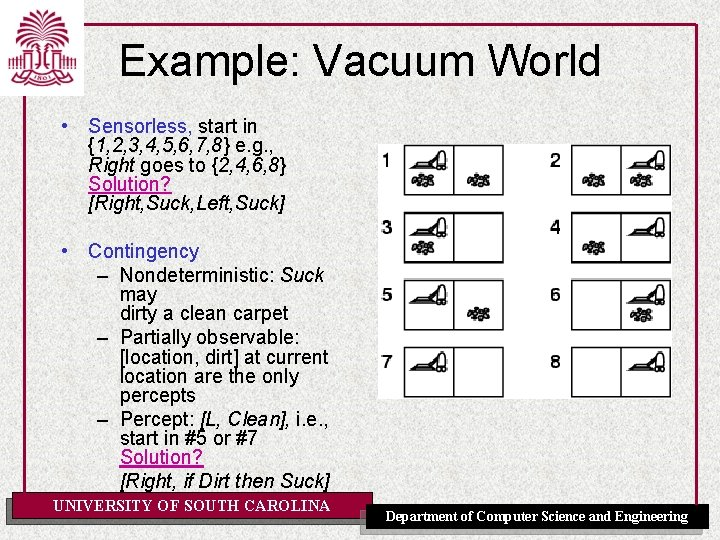Example: Vacuum World • Sensorless, start in {1, 2, 3, 4, 5, 6, 7, 8} e. g. , Right goes to {2, 4, 6, 8} Solution? [Right, Suck, Left, Suck] • Contingency – Nondeterministic: Suck may dirty a clean carpet – Partially observable: [location, dirt] at current location are the only percepts – Percept: [L, Clean], i. e. , start in #5 or #7 Solution? [Right, if Dirt then Suck] UNIVERSITY OF SOUTH CAROLINA Department of Computer Science and Engineering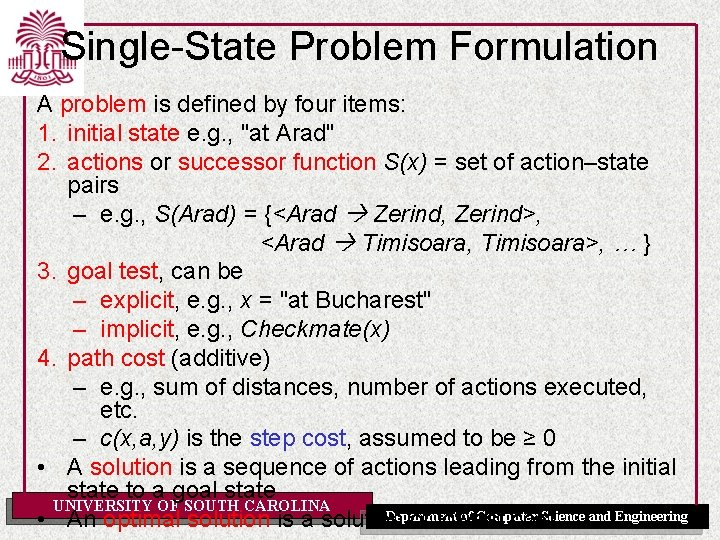Single-State Problem Formulation A problem is defined by four items: 1. initial state e. g. , "at Arad" 2. actions or successor function S(x) = set of action–state pairs – e. g. , S(Arad) = {<Arad Zerind, Zerind>, <Arad Timisoara, Timisoara>, … } 3. goal test, can be – explicit, e. g. , x = "at Bucharest" – implicit, e. g. , Checkmate(x) 4. path cost (additive) – e. g. , sum of distances, number of actions executed, etc. – c(x, a, y) is the step cost, assumed to be ≥ 0 • A solution is a sequence of actions leading from the initial state to a goal state UNIVERSITY OF SOUTH CAROLINA Department of Computer Science and Engineering • An optimal solution is a solution of lowest costSelecting a State Space • Real world is absurdly complex state space must be abstracted for problem solving • (Abstract) state = set of real states • (Abstract) action = complex combination of real actions – e. g. , “Arad Zerind” represents a complex set of possible routes, detours, rest stops, etc. • For guaranteed realizability, any real state “in Arad” must get to some real state “in Zerind” • (Abstract) solution = – set of real paths that are solutions in the real world • Each abstract action should be “easier” than the UNIVERSITY OF SOUTH CAROLINA Department of Computer Science and Engineering original problemVacuum World State Space Graph • • • States? Initial state? Actions? Goal test? Path cost? UNIVERSITY OF SOUTH CAROLINA Department of Computer Science and Engineering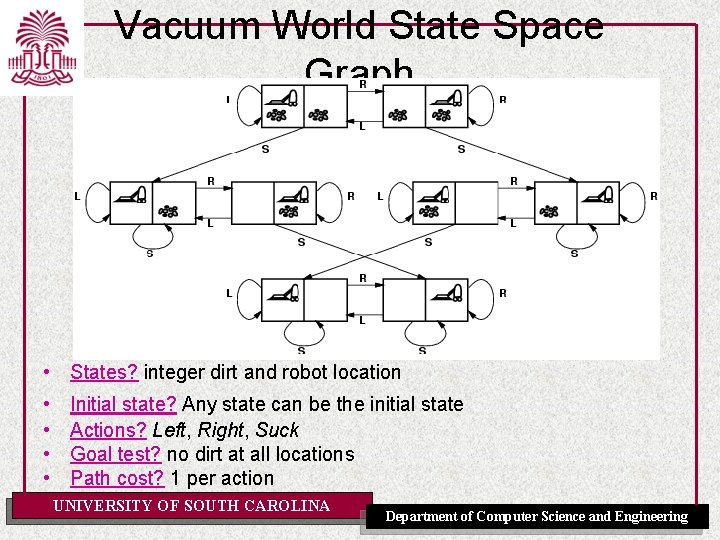Vacuum World State Space Graph • States? integer dirt and robot location • • Initial state? Any state can be the initial state Actions? Left, Right, Suck Goal test? no dirt at all locations Path cost? 1 per action UNIVERSITY OF SOUTH CAROLINA Department of Computer Science and Engineering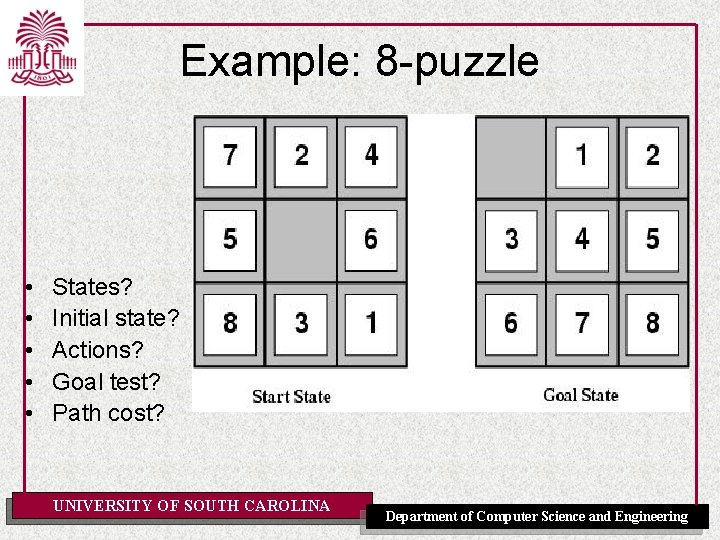Example: 8 -puzzle • • • States? Initial state? Actions? Goal test? Path cost? UNIVERSITY OF SOUTH CAROLINA Department of Computer Science and Engineering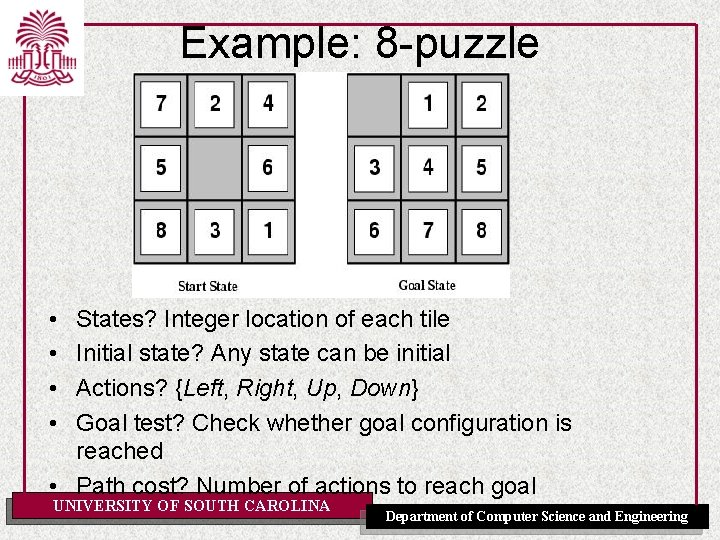Example: 8 -puzzle • • States? Integer location of each tile Initial state? Any state can be initial Actions? {Left, Right, Up, Down} Goal test? Check whether goal configuration is reached • Path cost? Number of actions to reach goal UNIVERSITY OF SOUTH CAROLINA Department of Computer Science and Engineering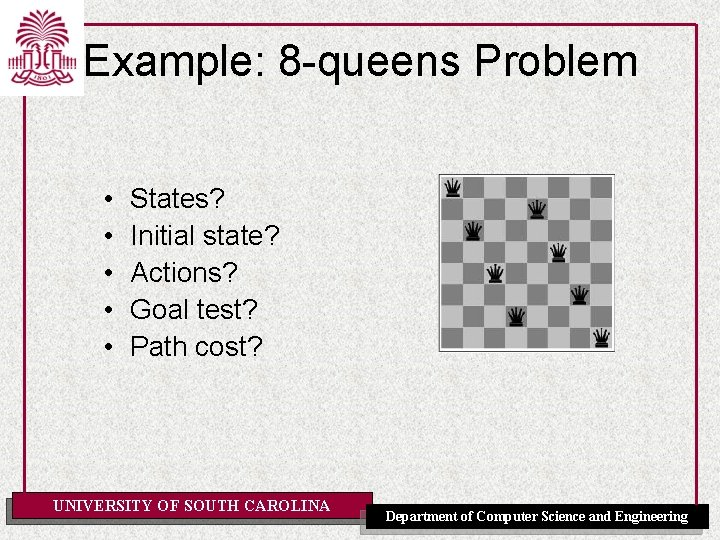Example: 8 -queens Problem • • • States? Initial state? Actions? Goal test? Path cost? UNIVERSITY OF SOUTH CAROLINA Department of Computer Science and EngineeringExample: 8 -queens Problem Incremental formulation vs. complete-state formulation • States? • Initial state? • Actions? • Goal test? • Path cost? UNIVERSITY OF SOUTH CAROLINA Department of Computer Science and EngineeringExample: 8 -queens Problem Incremental formulation • States? Any arrangement of 0 to 8 queens on the board • Initial state? No queens • Actions? Add queen in empty square • Goal test? 8 queens on board and none attacked • Path cost? None 3 x 1014 possible sequences to investigate UNIVERSITY OF SOUTH CAROLINA Department of Computer Science and EngineeringExample: 8 -queens Problem Incremental formulation (alternative) • States? n (0≤ n≤ 8) queens on the board, one per column in the n leftmost columns with no queen attacking another. • Actions? Add queen in leftmost empty column such that is not attacking other queens 2057 possible sequences to investigate; Yet makes no difference when n=100 UNIVERSITY OF SOUTH CAROLINA Department of Computer Science and EngineeringSome Real-World Problems • • Route Finding Touring Traveling Salesperson VLSI Layout – One-dimensional placement – Cell layout – Channel routing Robot navigation Automatic Assembly Sequencing Internet searching Various problems in bioinformatics UNIVERSITY OF SOUTH CAROLINA Department of Computer Science and EngineeringExample: Robotic Assembly • • • States? Initial state? Actions? Goal test? Path cost? UNIVERSITY OF SOUTH CAROLINA Department of Computer Science and Engineering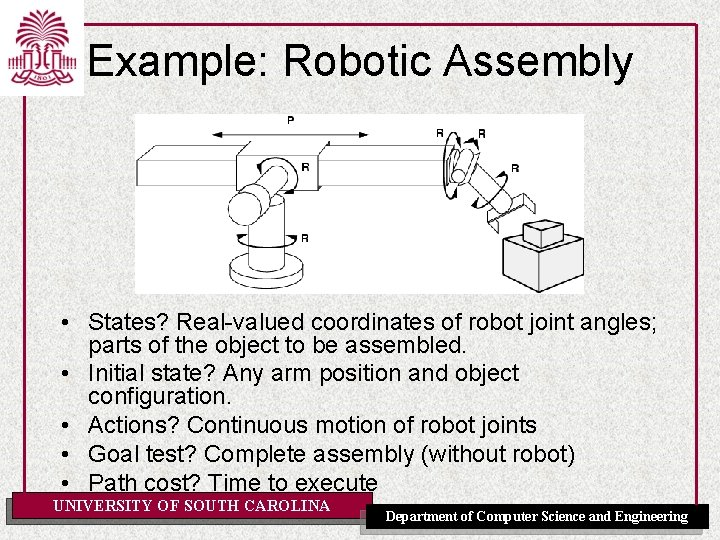Example: Robotic Assembly • States? Real-valued coordinates of robot joint angles; parts of the object to be assembled. • Initial state? Any arm position and object configuration. • Actions? Continuous motion of robot joints • Goal test? Complete assembly (without robot) • Path cost? Time to execute UNIVERSITY OF SOUTH CAROLINA Department of Computer Science and Engineering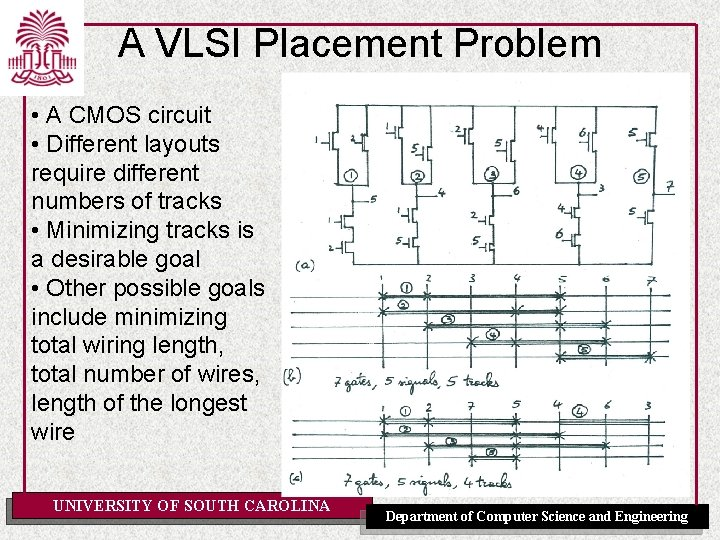A VLSI Placement Problem • A CMOS circuit • Different layouts require different numbers of tracks • Minimizing tracks is a desirable goal • Other possible goals include minimizing total wiring length, total number of wires, length of the longest wire UNIVERSITY OF SOUTH CAROLINA Department of Computer Science and Engineering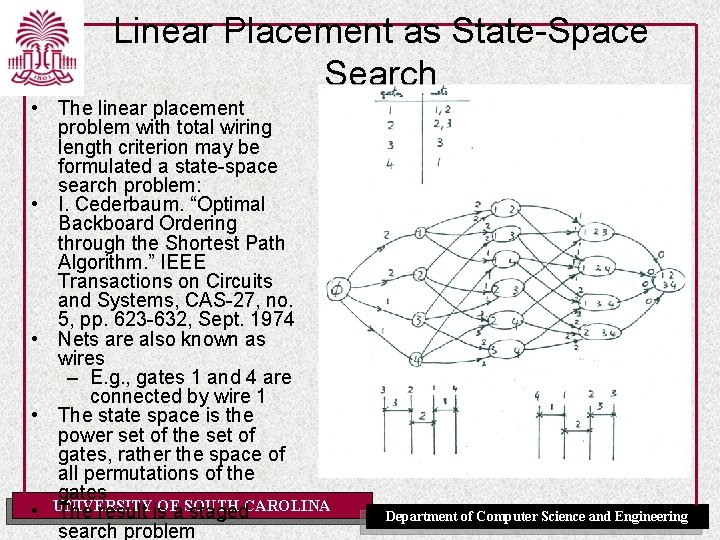Linear Placement as State-Space Search • The linear placement problem with total wiring length criterion may be formulated a state-space search problem: • I. Cederbaum. “Optimal Backboard Ordering through the Shortest Path Algorithm. ” IEEE Transactions on Circuits and Systems, CAS-27, no. 5, pp. 623 -632, Sept. 1974 • Nets are also known as wires – E. g. , gates 1 and 4 are connected by wire 1 • The state space is the power set of the set of gates, rather the space of all permutations of the gates UNIVERSITY • The result is. OFa. SOUTH staged. CAROLINA search problem Department of Computer Science and Engineering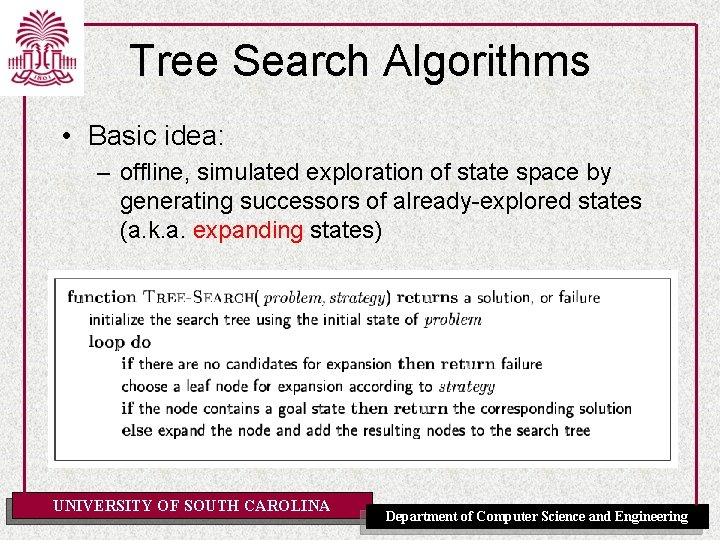Tree Search Algorithms • Basic idea: – offline, simulated exploration of state space by generating successors of already-explored states (a. k. a. expanding states) UNIVERSITY OF SOUTH CAROLINA Department of Computer Science and Engineering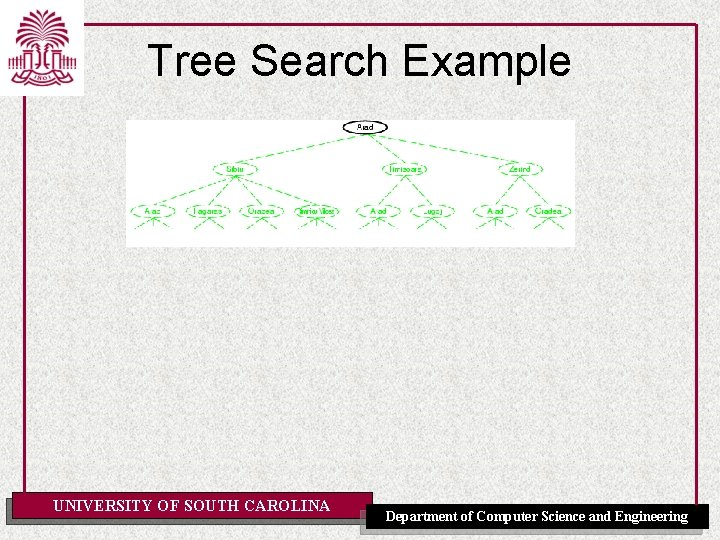Tree Search Example UNIVERSITY OF SOUTH CAROLINA Department of Computer Science and Engineering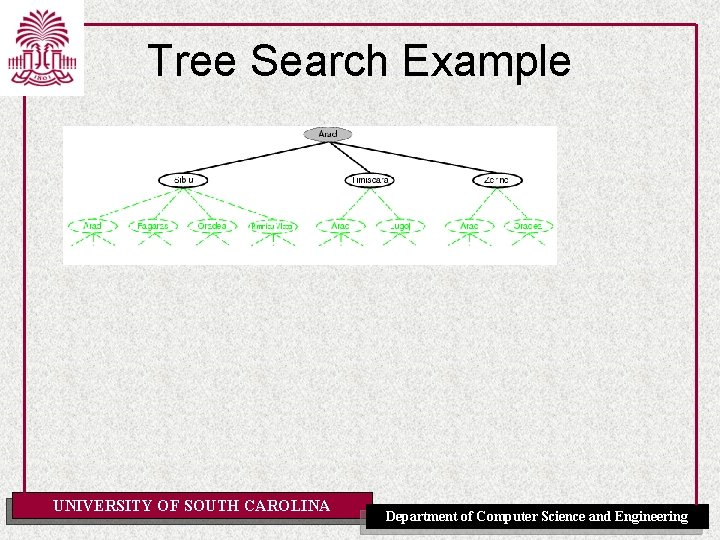Tree Search Example UNIVERSITY OF SOUTH CAROLINA Department of Computer Science and Engineering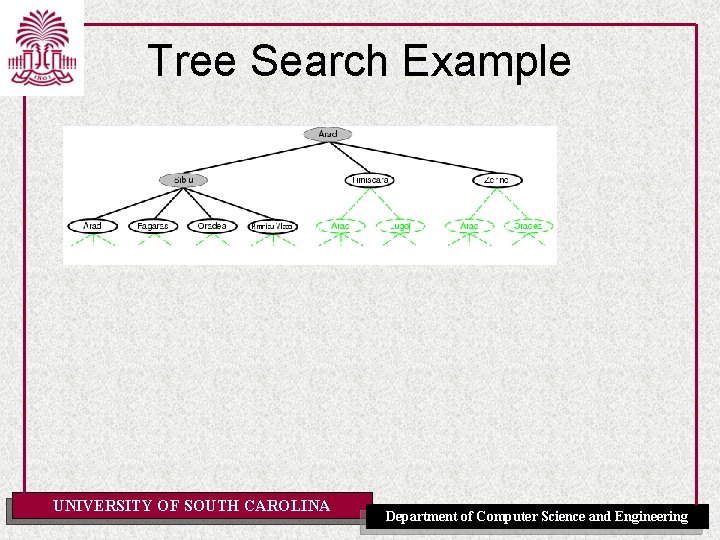Tree Search Example UNIVERSITY OF SOUTH CAROLINA Department of Computer Science and Engineering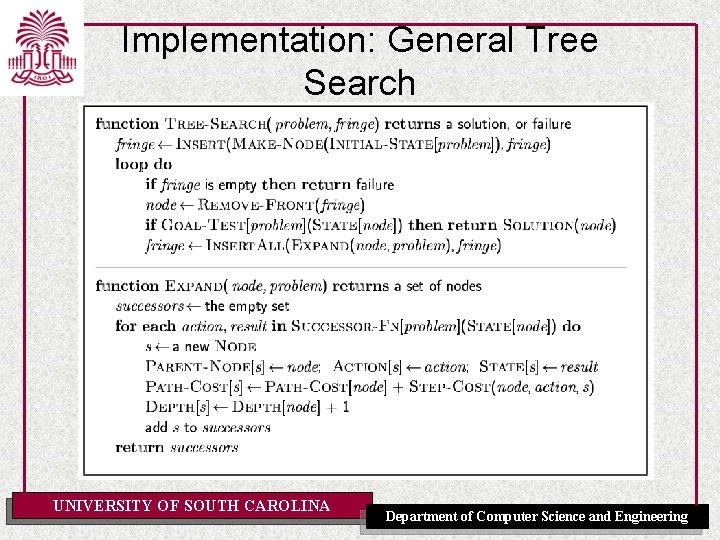Implementation: General Tree Search UNIVERSITY OF SOUTH CAROLINA Department of Computer Science and Engineering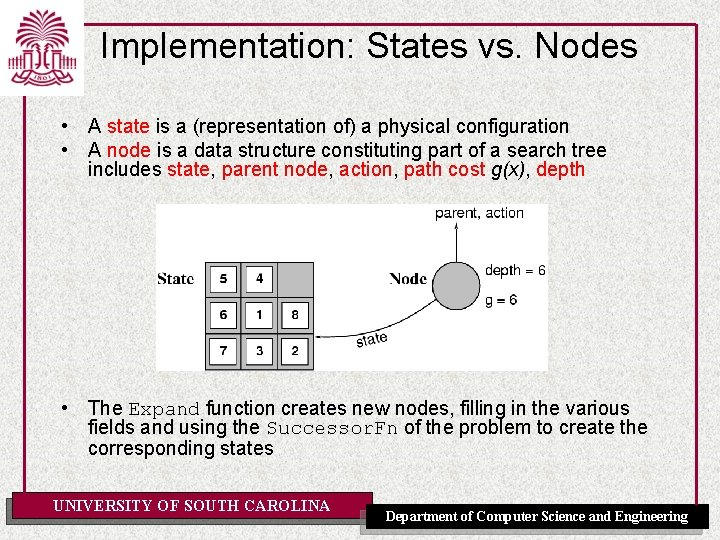Implementation: States vs. Nodes • A state is a (representation of) a physical configuration • A node is a data structure constituting part of a search tree includes state, parent node, action, path cost g(x), depth • The Expand function creates new nodes, filling in the various fields and using the Successor. Fn of the problem to create the corresponding states UNIVERSITY OF SOUTH CAROLINA Department of Computer Science and EngineeringSearch Strategies • A search strategy is defined by picking the order of node expansion • Strategies are evaluated along the following dimensions: – completeness: does it always find a solution if one exists? – time complexity: number of nodes generated (or: expanded) – space complexity: maximum number of nodes in memory – optimality: does it always find a least-cost solution? • Time and space complexity are measured in terms of – b: maximum branching factor of the search tree – d: depth of the least-cost solution UNIVERSITY OF SOUTH CAROLINA of Computer Science be and Engineering – m: maximum depth of the. Department state space (may ∞)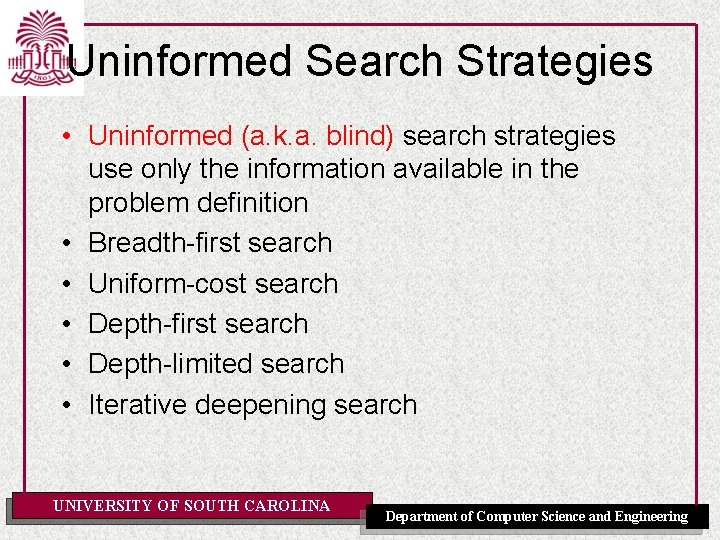Uninformed Search Strategies • Uninformed (a. k. a. blind) search strategies use only the information available in the problem definition • Breadth-first search • Uniform-cost search • Depth-first search • Depth-limited search • Iterative deepening search UNIVERSITY OF SOUTH CAROLINA Department of Computer Science and Engineering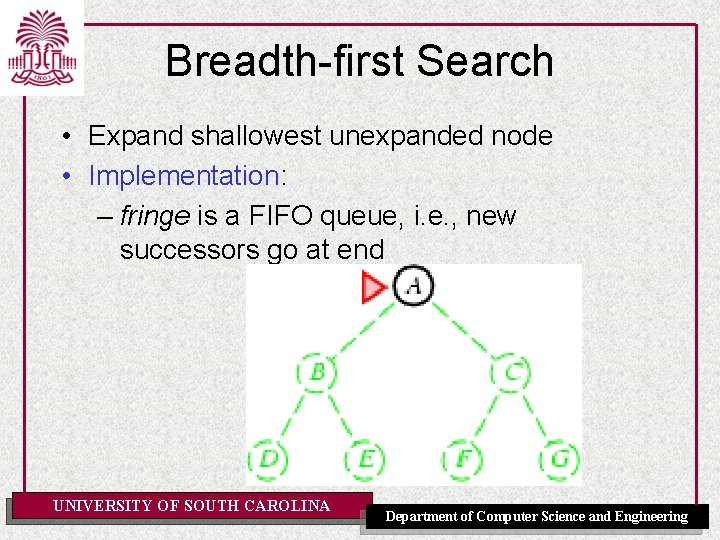Breadth-first Search • Expand shallowest unexpanded node • Implementation: – fringe is a FIFO queue, i. e. , new successors go at end UNIVERSITY OF SOUTH CAROLINA Department of Computer Science and EngineeringBreadth-first Search • Expand shallowest unexpanded node • Implementation: – fringe is a FIFO queue, i. e. , new successors go at end UNIVERSITY OF SOUTH CAROLINA Department of Computer Science and Engineering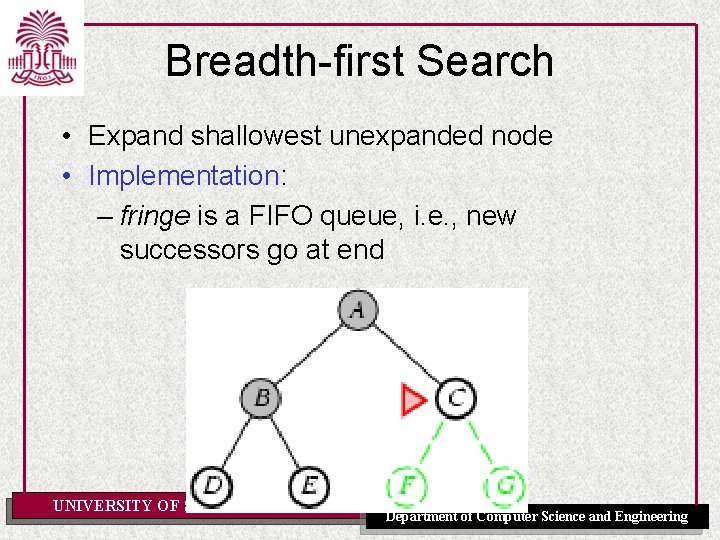Breadth-first Search • Expand shallowest unexpanded node • Implementation: – fringe is a FIFO queue, i. e. , new successors go at end UNIVERSITY OF SOUTH CAROLINA Department of Computer Science and Engineering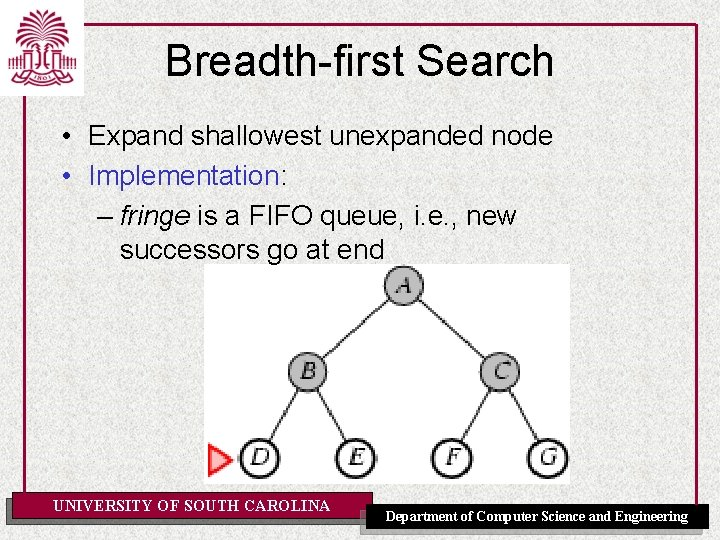Breadth-first Search • Expand shallowest unexpanded node • Implementation: – fringe is a FIFO queue, i. e. , new successors go at end UNIVERSITY OF SOUTH CAROLINA Department of Computer Science and Engineering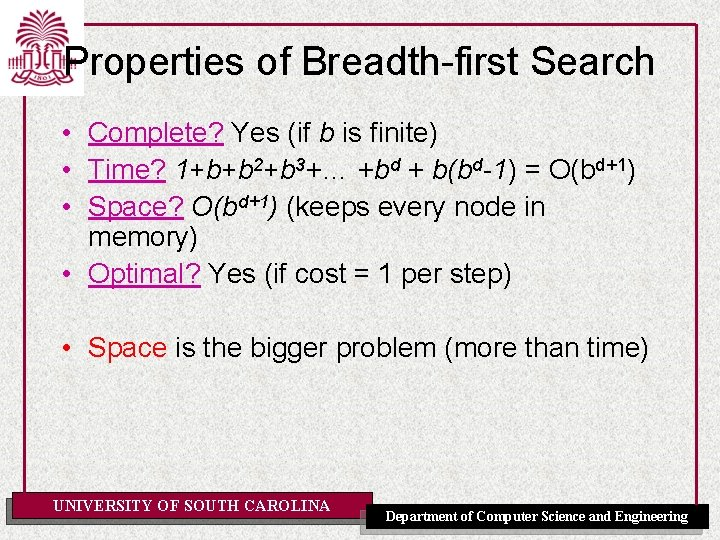Properties of Breadth-first Search • Complete? Yes (if b is finite) • Time? 1+b+b 2+b 3+… +bd + b(bd-1) = O(bd+1) • Space? O(bd+1) (keeps every node in memory) • Optimal? Yes (if cost = 1 per step) • Space is the bigger problem (more than time) UNIVERSITY OF SOUTH CAROLINA Department of Computer Science and Engineering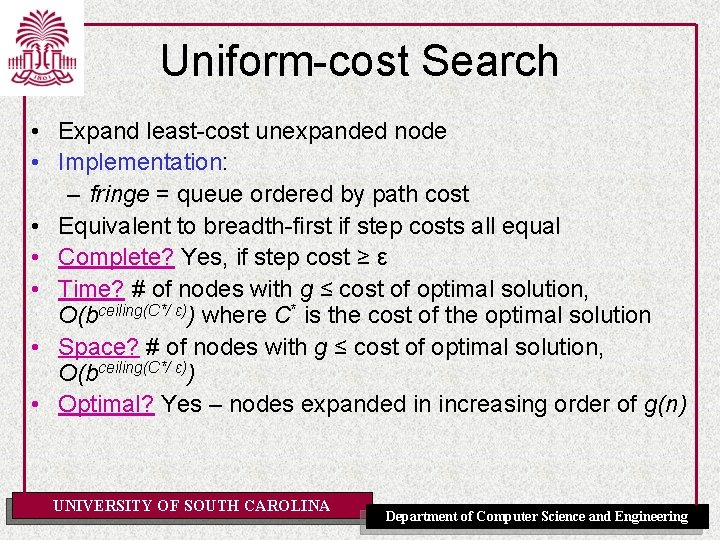Uniform-cost Search • Expand least-cost unexpanded node • Implementation: – fringe = queue ordered by path cost • Equivalent to breadth-first if step costs all equal • Complete? Yes, if step cost ≥ ε • Time? # of nodes with g ≤ cost of optimal solution, O(bceiling(C*/ ε)) where C* is the cost of the optimal solution • Space? # of nodes with g ≤ cost of optimal solution, O(bceiling(C*/ ε)) • Optimal? Yes – nodes expanded in increasing order of g(n) UNIVERSITY OF SOUTH CAROLINA Department of Computer Science and EngineeringDepth-first Search • Expand deepest unexpanded node • Implementation: – fringe = LIFO queue, i. e. , put successors at front UNIVERSITY OF SOUTH CAROLINA Department of Computer Science and Engineering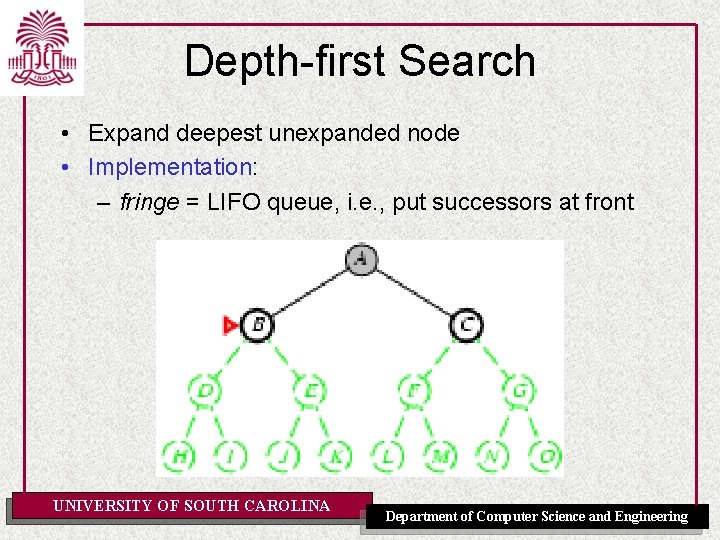Depth-first Search • Expand deepest unexpanded node • Implementation: – fringe = LIFO queue, i. e. , put successors at front UNIVERSITY OF SOUTH CAROLINA Department of Computer Science and EngineeringDepth-first Search • Expand deepest unexpanded node • Implementation: – fringe = LIFO queue, i. e. , put successors at front UNIVERSITY OF SOUTH CAROLINA Department of Computer Science and Engineering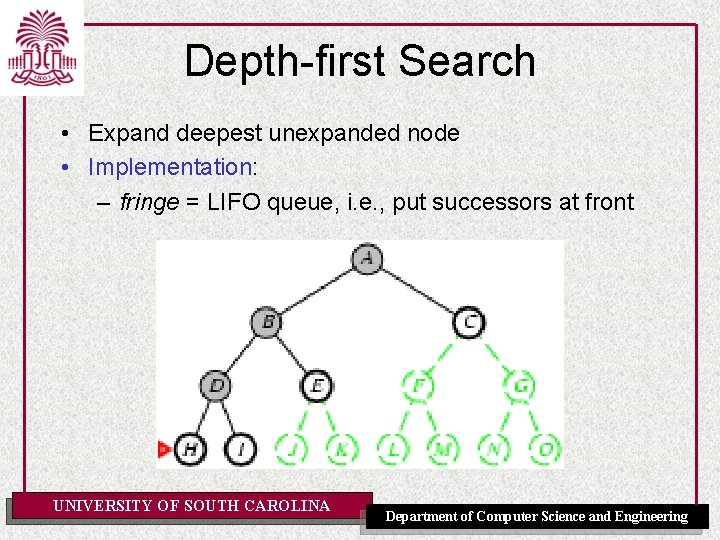Depth-first Search • Expand deepest unexpanded node • Implementation: – fringe = LIFO queue, i. e. , put successors at front UNIVERSITY OF SOUTH CAROLINA Department of Computer Science and EngineeringDepth-first Search • Expand deepest unexpanded node • Implementation: – fringe = LIFO queue, i. e. , put successors at front UNIVERSITY OF SOUTH CAROLINA Department of Computer Science and EngineeringDepth-first Search • Expand deepest unexpanded node • Implementation: – fringe = LIFO queue, i. e. , put successors at front UNIVERSITY OF SOUTH CAROLINA Department of Computer Science and Engineering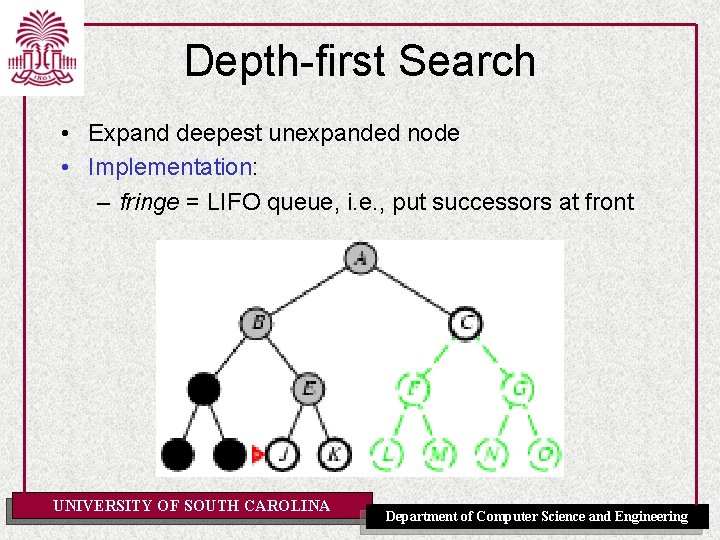Depth-first Search • Expand deepest unexpanded node • Implementation: – fringe = LIFO queue, i. e. , put successors at front UNIVERSITY OF SOUTH CAROLINA Department of Computer Science and Engineering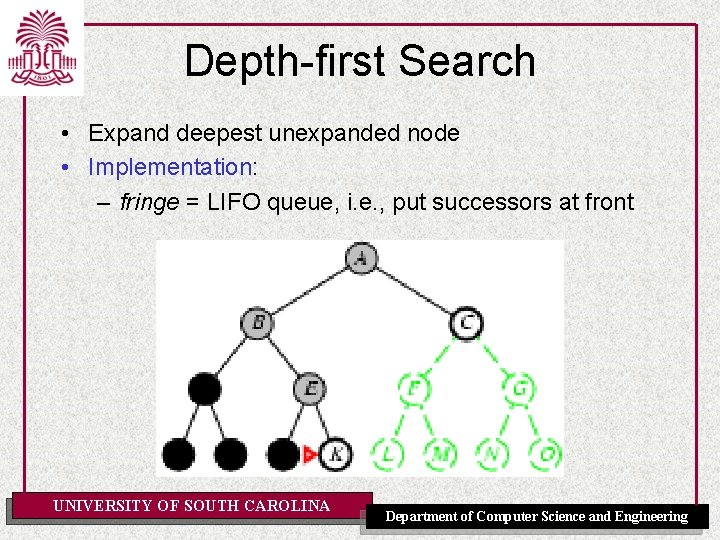Depth-first Search • Expand deepest unexpanded node • Implementation: – fringe = LIFO queue, i. e. , put successors at front UNIVERSITY OF SOUTH CAROLINA Department of Computer Science and Engineering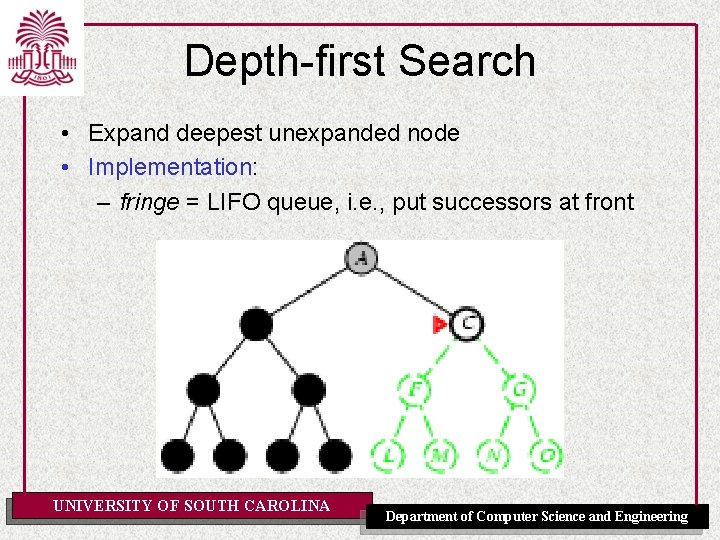Depth-first Search • Expand deepest unexpanded node • Implementation: – fringe = LIFO queue, i. e. , put successors at front UNIVERSITY OF SOUTH CAROLINA Department of Computer Science and EngineeringDepth-first Search • Expand deepest unexpanded node • Implementation: – fringe = LIFO queue, i. e. , put successors at front UNIVERSITY OF SOUTH CAROLINA Department of Computer Science and Engineering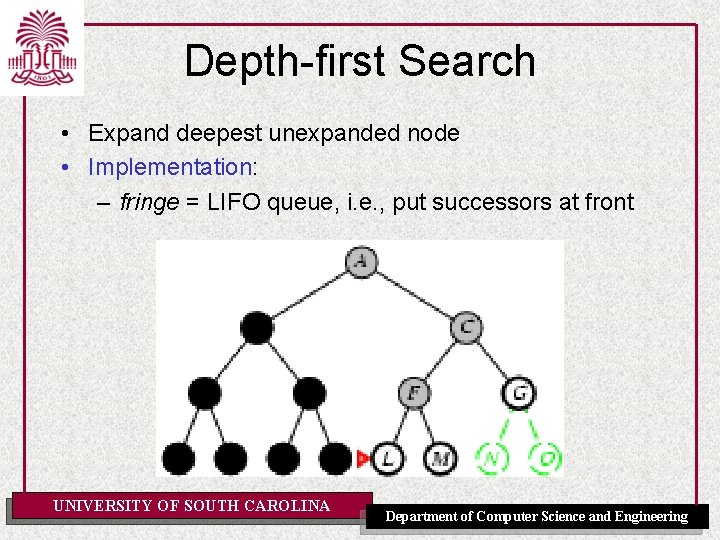Depth-first Search • Expand deepest unexpanded node • Implementation: – fringe = LIFO queue, i. e. , put successors at front UNIVERSITY OF SOUTH CAROLINA Department of Computer Science and Engineering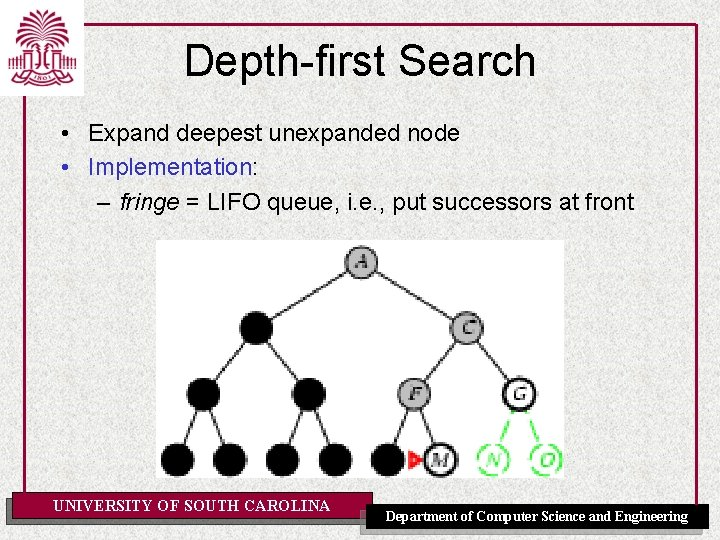Depth-first Search • Expand deepest unexpanded node • Implementation: – fringe = LIFO queue, i. e. , put successors at front UNIVERSITY OF SOUTH CAROLINA Department of Computer Science and Engineering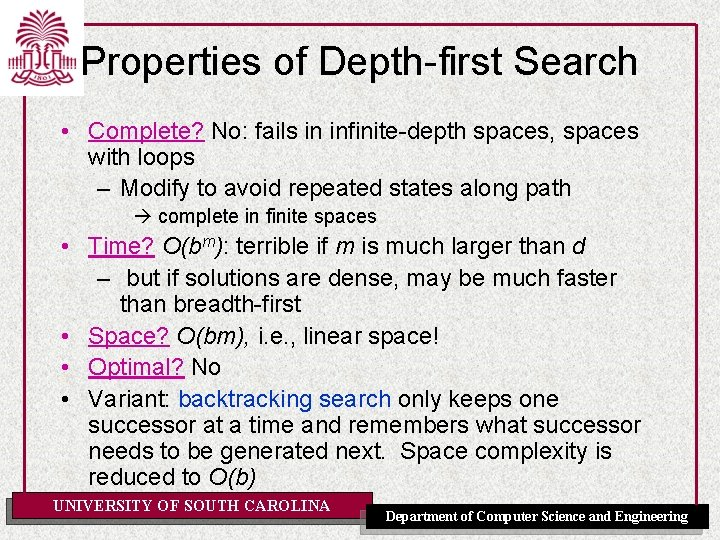Properties of Depth-first Search • Complete? No: fails in infinite-depth spaces, spaces with loops – Modify to avoid repeated states along path complete in finite spaces • Time? O(bm): terrible if m is much larger than d – but if solutions are dense, may be much faster than breadth-first • Space? O(bm), i. e. , linear space! • Optimal? No • Variant: backtracking search only keeps one successor at a time and remembers what successor needs to be generated next. Space complexity is reduced to O(b) UNIVERSITY OF SOUTH CAROLINA Department of Computer Science and EngineeringDepth-limited Search = depth-first search with depth limit l, i. e. , nodes at depth l have no successors • Recursive implementation: UNIVERSITY OF SOUTH CAROLINA Department of Computer Science and Engineering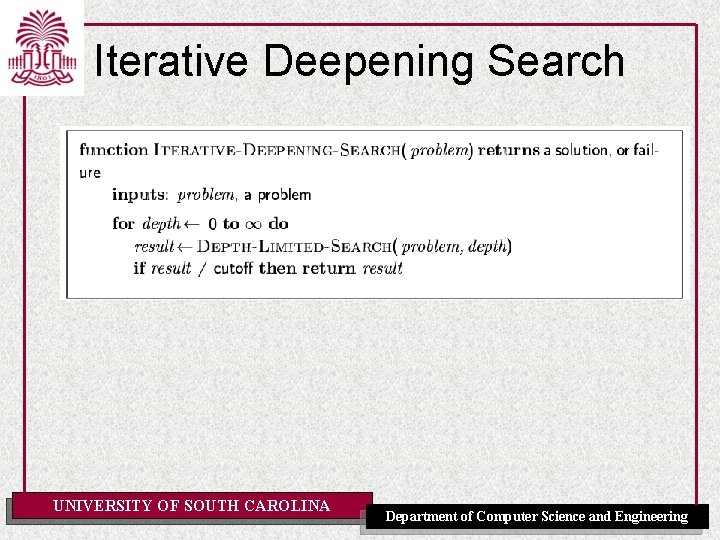Iterative Deepening Search UNIVERSITY OF SOUTH CAROLINA Department of Computer Science and Engineering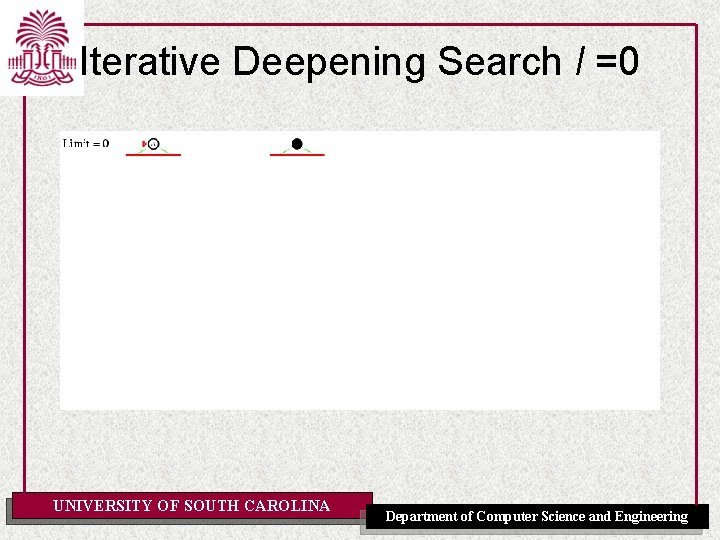Iterative Deepening Search l =0 UNIVERSITY OF SOUTH CAROLINA Department of Computer Science and Engineering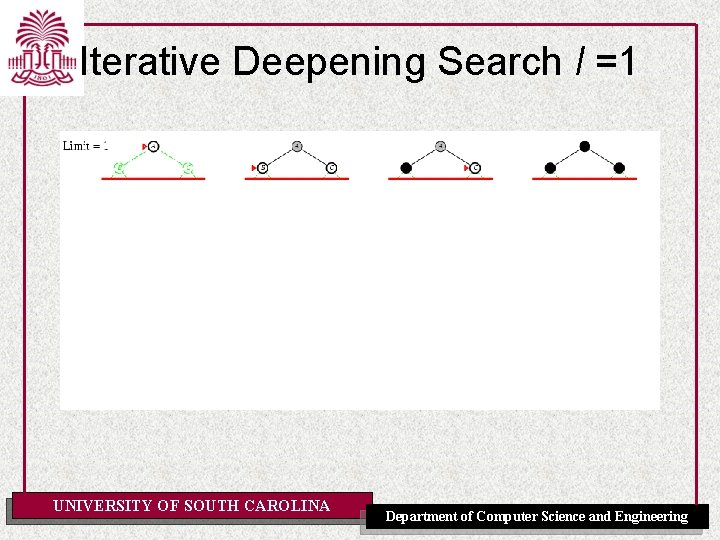Iterative Deepening Search l =1 UNIVERSITY OF SOUTH CAROLINA Department of Computer Science and Engineering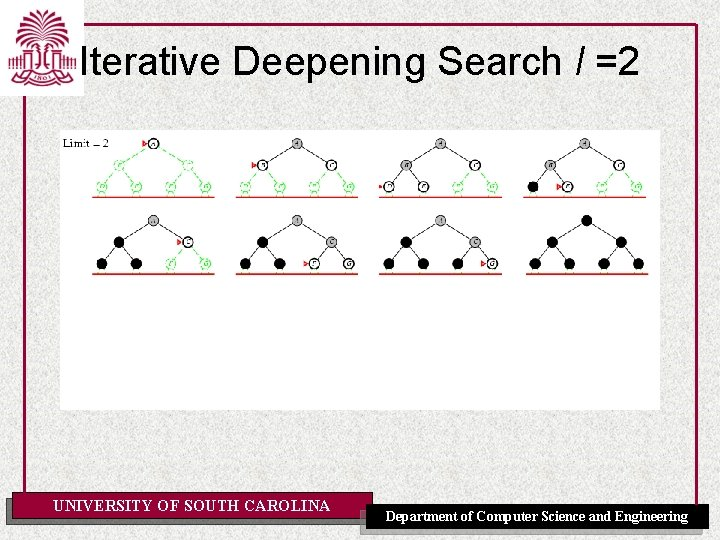Iterative Deepening Search l =2 UNIVERSITY OF SOUTH CAROLINA Department of Computer Science and Engineering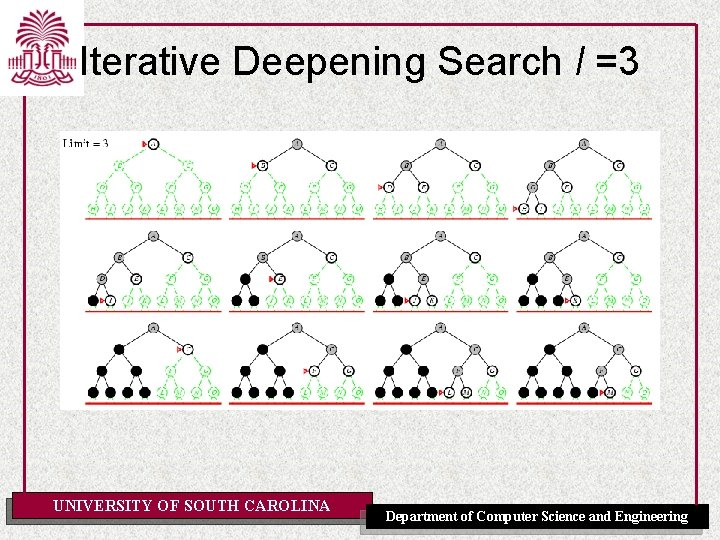Iterative Deepening Search l =3 UNIVERSITY OF SOUTH CAROLINA Department of Computer Science and EngineeringIterative Deepening Search • Number of nodes generated in a depth-limited search to depth d with branching factor b: NDLS = b 0 + b 1 + b 2 + … + bd-2 + bd-1 + bd • Number of nodes generated in an iterative deepening search to depth d with branching factor b: NIDS = (d+1)b 0 + d b 1 + (d-1)b 2 + … + 3 bd-2 +2 bd-1 + 1 bd • For b = 10, d = 5, – NDLS = 1 + 100 + 1, 000 + 100, 000 = 111, 111 – NIDS = 6 + 50 + 400 + 3, 000 + 20, 000 + 100, 000 = 123, 456 • Overhead = (123, 456 - 111, 111)/111, 111 = 11% UNIVERSITY OF SOUTH CAROLINA Department of Computer Science and EngineeringProperties of Iterative Deepening Search • Complete? Yes • Time? (d+1)b 0 + d b 1 + (d-1)b 2 + … + bd = O(bd) • Space? O(bd) • Optimal? Yes, if step cost = 1 UNIVERSITY OF SOUTH CAROLINA Department of Computer Science and Engineering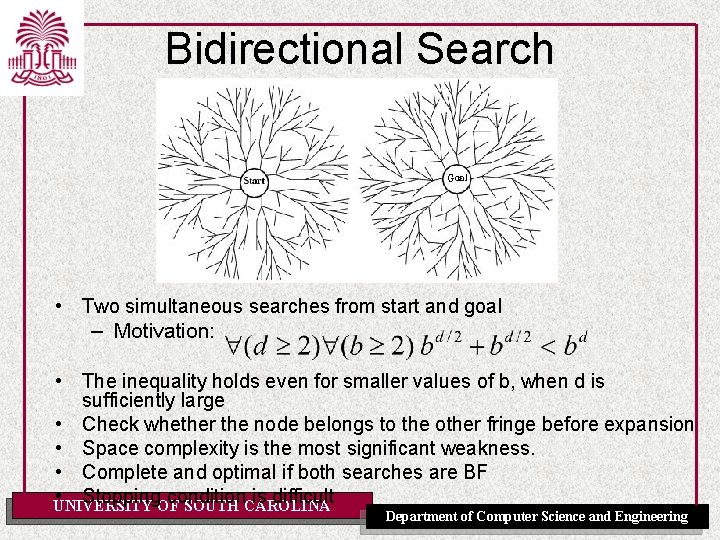Bidirectional Search • Two simultaneous searches from start and goal – Motivation: • The inequality holds even for smaller values of b, when d is sufficiently large • Check whether the node belongs to the other fringe before expansion • Space complexity is the most significant weakness. • Complete and optimal if both searches are BF • Stopping condition is difficult UNIVERSITY OF SOUTH CAROLINA Department of Computer Science and Engineering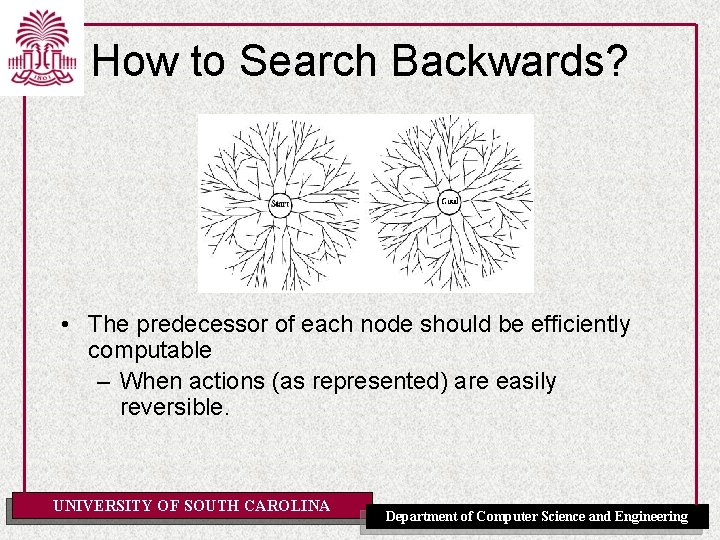How to Search Backwards? • The predecessor of each node should be efficiently computable – When actions (as represented) are easily reversible. UNIVERSITY OF SOUTH CAROLINA Department of Computer Science and Engineering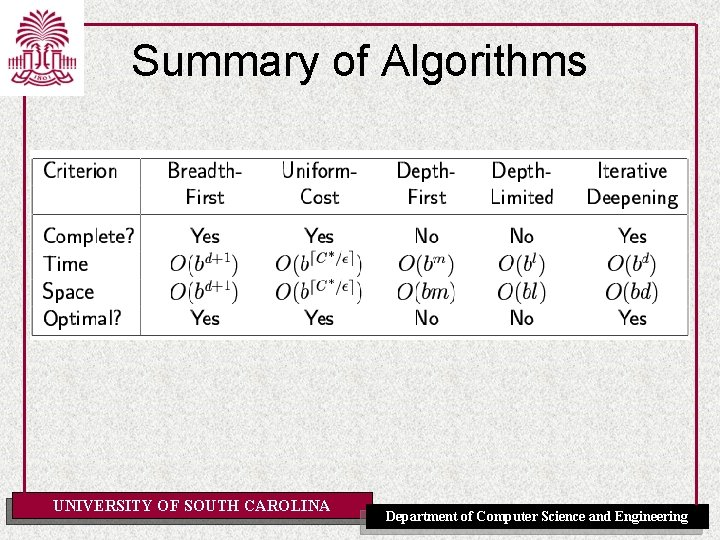Summary of Algorithms UNIVERSITY OF SOUTH CAROLINA Department of Computer Science and Engineering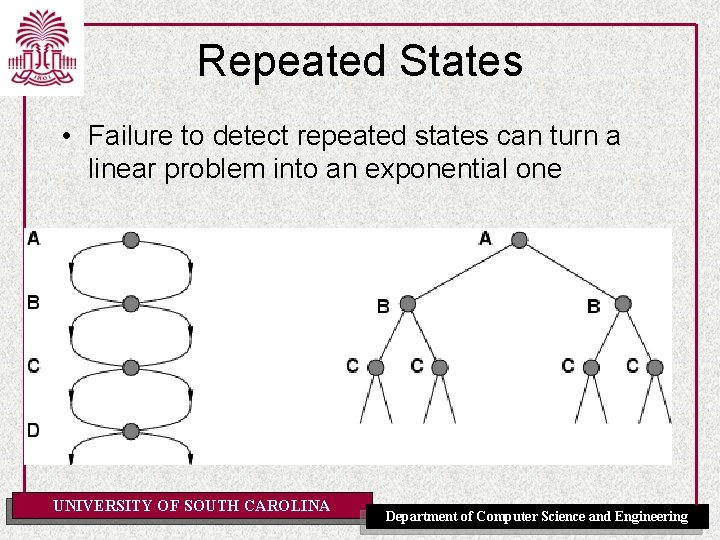Repeated States • Failure to detect repeated states can turn a linear problem into an exponential one UNIVERSITY OF SOUTH CAROLINA Department of Computer Science and Engineering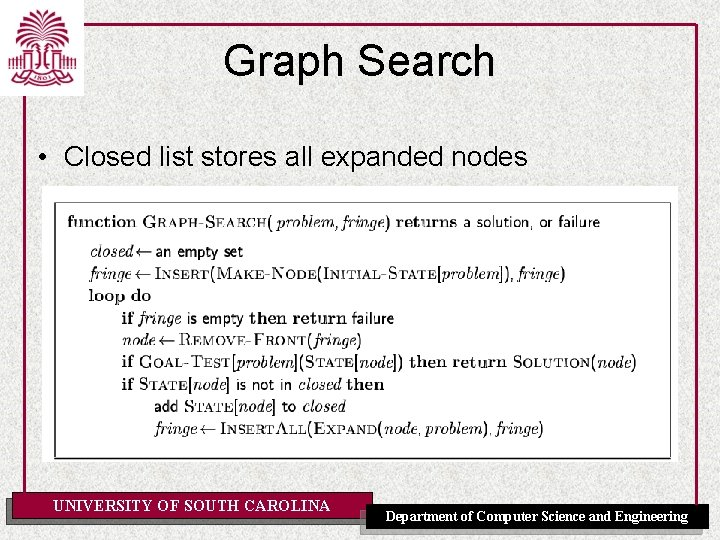Graph Search • Closed list stores all expanded nodes UNIVERSITY OF SOUTH CAROLINA Department of Computer Science and Engineering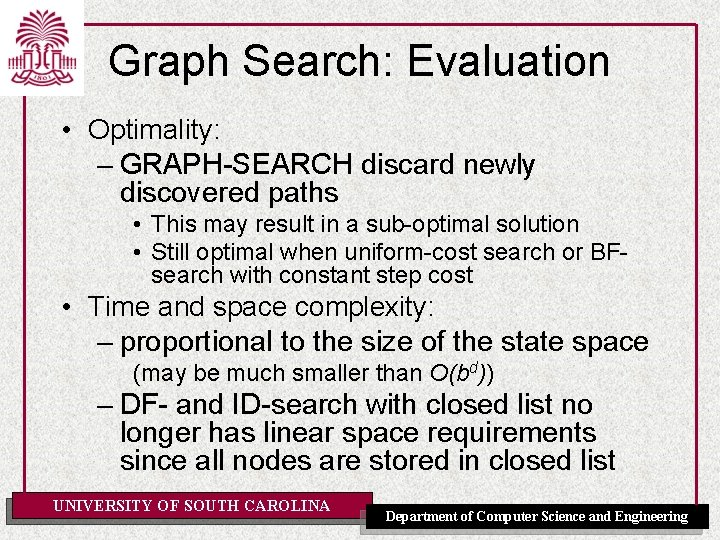Graph Search: Evaluation • Optimality: – GRAPH-SEARCH discard newly discovered paths • This may result in a sub-optimal solution • Still optimal when uniform-cost search or BFsearch with constant step cost • Time and space complexity: – proportional to the size of the state space (may be much smaller than O(bd)) – DF- and ID-search with closed list no longer has linear space requirements since all nodes are stored in closed list UNIVERSITY OF SOUTH CAROLINA Department of Computer Science and Engineering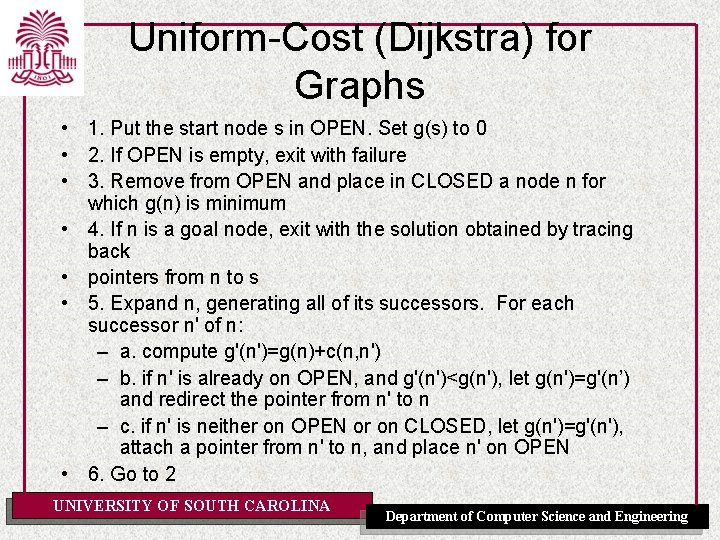Uniform-Cost (Dijkstra) for Graphs • 1. Put the start node s in OPEN. Set g(s) to 0 • 2. If OPEN is empty, exit with failure • 3. Remove from OPEN and place in CLOSED a node n for which g(n) is minimum • 4. If n is a goal node, exit with the solution obtained by tracing back • pointers from n to s • 5. Expand n, generating all of its successors. For each successor n' of n: – a. compute g'(n')=g(n)+c(n, n') – b. if n' is already on OPEN, and g'(n')<g(n'), let g(n')=g'(n’) and redirect the pointer from n' to n – c. if n' is neither on OPEN or on CLOSED, let g(n')=g'(n'), attach a pointer from n' to n, and place n' on OPEN • 6. Go to 2 UNIVERSITY OF SOUTH CAROLINA Department of Computer Science and Engineering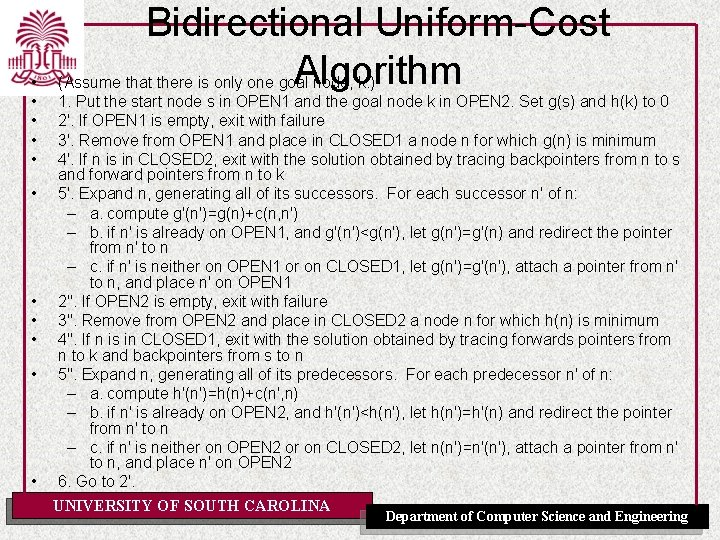• • • Bidirectional Uniform-Cost Algorithm (Assume that there is only one goal node, k. ) 1. Put the start node s in OPEN 1 and the goal node k in OPEN 2. Set g(s) and h(k) to 0 2'. If OPEN 1 is empty, exit with failure 3'. Remove from OPEN 1 and place in CLOSED 1 a node n for which g(n) is minimum 4'. If n is in CLOSED 2, exit with the solution obtained by tracing backpointers from n to s and forward pointers from n to k 5'. Expand n, generating all of its successors. For each successor n' of n: – a. compute g'(n')=g(n)+c(n, n') – b. if n' is already on OPEN 1, and g'(n')<g(n'), let g(n')=g'(n) and redirect the pointer from n' to n – c. if n' is neither on OPEN 1 or on CLOSED 1, let g(n')=g'(n'), attach a pointer from n' to n, and place n' on OPEN 1 2". If OPEN 2 is empty, exit with failure 3". Remove from OPEN 2 and place in CLOSED 2 a node n for which h(n) is minimum 4". If n is in CLOSED 1, exit with the solution obtained by tracing forwards pointers from n to k and backpointers from s to n 5". Expand n, generating all of its predecessors. For each predecessor n' of n: – a. compute h'(n')=h(n)+c(n', n) – b. if n' is already on OPEN 2, and h'(n')<h(n'), let h(n')=h'(n) and redirect the pointer from n' to n – c. if n' is neither on OPEN 2 or on CLOSED 2, let n(n')=n'(n'), attach a pointer from n' to n, and place n' on OPEN 2 6. Go to 2'. UNIVERSITY OF SOUTH CAROLINA Department of Computer Science and EngineeringSummary • Problem formulation usually requires abstracting away real-world details to define a state space that can feasibly be explored • Variety of uninformed search strategies • Iterative deepening search uses only linear space and not much more time than other uninformed algorithms – It is the preferred blind search method for trees when there is a large search space, the length of the solution is unknown, and the cost of each action is the same UNIVERSITY OF SOUTH CAROLINA Department of Computer Science and EngineeringSearch with Partial Information • Previous assumption: – Environment is fully observable – Environment is deterministic – Agent knows the effects of its actions What if knowledge of states or actions is incomplete? UNIVERSITY OF SOUTH CAROLINA Department of Computer Science and Engineering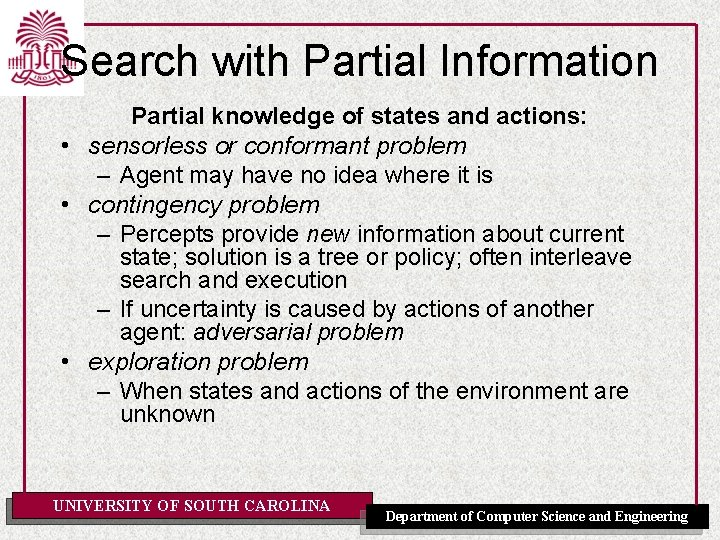Search with Partial Information Partial knowledge of states and actions: • sensorless or conformant problem – Agent may have no idea where it is • contingency problem – Percepts provide new information about current state; solution is a tree or policy; often interleave search and execution – If uncertainty is caused by actions of another agent: adversarial problem • exploration problem – When states and actions of the environment are unknown UNIVERSITY OF SOUTH CAROLINA Department of Computer Science and EngineeringSensorless Vacuum World • Search space of belief states • Solution = belief state with all members goal states. • If S states then 2 S belief states. • Murphy’s law: – Suck can dirty a clear square. UNIVERSITY OF SOUTH CAROLINA Department of Computer Science and EngineeringSensorless Vacuum World • start in {1, 2, 3, 4, 5, 6, 7, 8} e. g Right goes to {2, 4, 6, 8}. Solution? – [Right, Suck, Left, Suck] • When the world is not fully observable: reason about a set of states that might be reached =belief state UNIVERSITY OF SOUTH CAROLINA Department of Computer Science and Engineering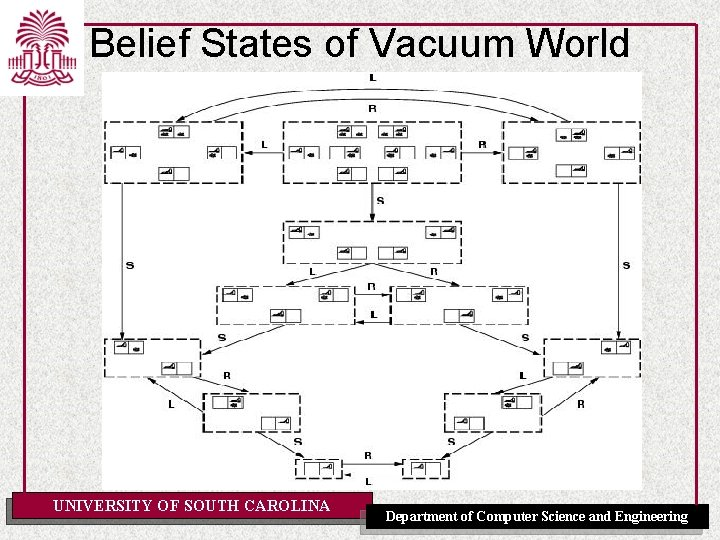Belief States of Vacuum World UNIVERSITY OF SOUTH CAROLINA Department of Computer Science and EngineeringContingency Problems • • • Contingency, start in {1, 3}. Murphy’s law, Suck can dirty a clean carpet. Local sensing: dirt, location only. – Percept = [L, Dirty] ={1, 3} – [Suck] = {5, 7} – [Right] ={6, 8} – [Suck] in {6}={8} (Success) – BUT [Suck] in {8} = failure Solution? – Belief-state: no fixed action sequence guarantees solution Relax requirement: – [Suck, Right, if [R, dirty] then Suck] – Select actions based on contingencies arising during execution. UNIVERSITY OF SOUTH CAROLINA Department of Computer Science and Engineering